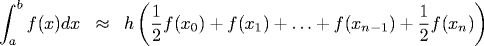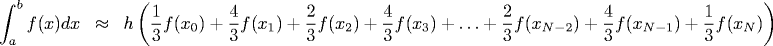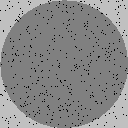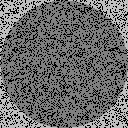# 9.3. NUMERICAL INTEGRATION

This section under major construction.

Midpoint rule. Goal: given continuous function f(x) of one variable, compute ∫ f(x) dx over interval from a to b. Estimate integral by M = (b-a) * f(c), where c = (a + b)/2.

Trapezoidal rule. Goal: given continuous function f(x) of one variable, compute ∫ f(x) dx over interval from a to b. TrapezoidalRule.java numerically integrates a function of one variable using the trapezoidal rule. We can estimate the integral of f(x) from a to b using the formula T = (b-a)/2 (f(a) + f(b)). Breaking the interval from a to b up into N equally spaced intervals (and combining common terms) we obtain the formula:where the interval [a, b] is broken up into N subintervals of uniform size h = (b - a) / N. Under certain technical conditions, if N is large then the formula above is a good estimate of the integral.

Simpson's rule. The trapezoidal rule is rarely used to integrate in practice. For smooth f, the midpoint rule is approximately twice as accurate as the trapezoidal rule, and the errors have different signs. By combining the two expressions, we obtain a more accurate estimate of f: S = 2/3*M + 1/3*T. This combination is known as Simpson's 1/3 rule. S = (b-a)/6 (f(a) + 4f(c) + f(b)), where c = (a + b)/2. Breaking the interval from a to b up into N equally spaced intervals (and combining common terms) we obtain the formula:where the interval [a, b] is broken up into N subintervals of uniform size h = (b - a) / (N - 1) and the 2/3 and 4/3 coefficients alternate throughout the interior. Here are some nice animations of numerical quadrature. Under certain technical conditions, if N is large then the formula above is a good estimate of the integral. The program SimpsonsRule.java numerically integrates x^4 log (x + sqrt(x^2 + 1)) from a = 0 to b = 2.

Program organization. To write a reusable intergration routine, we would like to be able to create a class TrapezoidalRule and pass an arbitrary continuous function to be integrated. One way of accomplishing this is to declare an interface for continuous functions that has a single method eval, which takes a real argument x and returns f(x).

 public interface ContinuousFunction { public double eval(double x); } 

Then, we could implement the error function as

 public class NormalPDF implements ContinuousFunction { private double mu; private double sigma; public NormalPDF(double mu, double sigma) { this.mu = mu; this.sigma = sigma; } public double eval(double x) { return Math.exp(- x * x / 2) / Math.sqrt(2 * Math.PI); // mu, sigma } } 

 Q1 = h/6 (f(a) + 4f(c) + f(b)), where c = (a + b)/2 [Simpson] Q2 = h/12 (f(a) + 4f(d) + 2f(c) + 4f(e) + f(b)), [Simpson over two subintervals] where d = (a + c)/2, e = (c + b)/2 Q = Q2 + (Q2 - Q1) / 15 [ 6th order Newton-Cotes / Weddle's Rule] 
The trapezoidal rule approximates the area under a curve by breaking up the interval into a fixed number of equally spaced subintervals, and approximating the area in each subinterval by a trapezoid. We can often obtain a more refined approximation by using a variable number of subintervals and choosing them according to the shape of the curve. In adaptive quadrature we estimate the area under a curve in the interval from a to b twice, one using Q1 and once using Q2. If these two estimates are sufficiently close, we estimate the area using Q = Q2 + (Q2 - Q1)/ 15. Otherwise, we divide up the interval into two equal subintervals from a to c and c to b, where c is the midpoint (a + b) / 2. We compute the area of each piece (recursively using adaptive quadrature) and sum the results. Program AdaptiveQuadrature.java implements this strategy.

If we are careful, we can save function evaluations from one iteration to the next and get away with just two function evaluations per recursive invocation. (See exercise.)

Our method fails spectacularly when integrating 1 / (3x - 1) from x = 0 to 1. Integral has a nonsingularity and our function will go into an infinite loop. An industrial strength implementation would diagnose such a situation and report an appropriate error message.

Monte Carlo integration. Theory based on the Law of Large Numbers. Estimating area of circle by rejection method. Dartboard. Throwing darts at Irish pub after several pints... Program Dartboard.java reads in a command line integer N, throws N darts uniformly distributed in the unit box, and plots the results. The pictures below show sample results for N = 1,000, 10,000, and 100,000.Alternate viewpoint: 2D Monte Carlo integration of f(x, y) = 1 if x2 + y2 ≤ 1. Show histogram of plot - normal with standard deviation.

To estimate the integral of f over a multi-dimensional volume V, we select N points x1, x2, ..., xN in the volume uniformly at random. Our estimate of the integral is the fraction of random points that fall below f multiplied by the volume = V <f>, where

  = 1/N * (f(x1) + ... + f(x2)) = 1/N * (f2(x1) + ... + f2(x2)) 

The fundamental theorem of Monte Carlo integration asserts that the integral of f over V equals V <f> +- V sqrt((<f2> - <f>2) / N). The key observation is that the error goes as 1 / sqrt(N). This means that you have to quadruple the number of simulations to double the accuracy of your approximation. However, there is no dependence on dimension! Not competitive in one or two dimensions, but its power shines in higher dimensions.

Center of mass of a torus. As an example (borrowed from p. 221 Numerical Recipes), suppose we want to estimate the weight and center of mass of the intersection of a torus and two planes, defined by points (x, y, z) satisfying

 z2 + (sqrt(x2 + y2) - 3)2 ≤ 1 x ≥ 1 y ≥ -3 

We must compute the integrals f(x, y, z) = ρ, fx(x, y, z) = xρ, fy(x, y, z) = yρ, and fz(x, y, z) = zρ. The center of mass is then (fx/f, fy/f, fz/f). To sample a point (x, y, z) uniformly from the torus, we use the rejection method. In this case, the torus in enclosed in the rectangular box with 1 ≤ x ≤ 4, -3 ≤ y ≤ 4, and -1 ≤ z ≤ 1. Program Torus.java takes a command line parameter N and computes these quantities using N sample points.

Gaussian cdf. Estimate the Gaussian cdf Phi(z) by generating random numbers from a Gaussian distribution and recording the fraction of values less than z. This is an example of importance sampling. Note: can't sample uniformly between -infinity and infinity anyway.

#### Exercises

1. Integrate f(x) = xx from x = 0 to x = 1.

Answer: 1 - 2-2 + 3-3 - 4-4 + ... = 0.78343...

2. Numerically integrate f(x) = e-x sin(8x2/3) from 0 to 2.
3. Integrate f(x) = sin(x2) from x = 0 to x = pi.
4. Integrate f(x) = cos(20 x2) from x = 0 to x = 1.
5. Use the trapezoidal rule to numerically integrate f(x) = 4 sqrt(1 - x2) from x = -1 to 1. Note slow convergence to true answer of xyz.
6. Write a program to compute the sine integral Si(x), which is defined as the integral of (sin t) / t from 0 to x.
7. Write a program to compute the Fresnel sine integral FresnelSi(x), which is defined as the integral of sin (π/2 t2) from 0 to x.
8. Use Monte Carlo integration to approximate the two dimensional integral of f(x, y) = x2 + 6xy + y2 over the unit circle (x2 + y2 ≤ 1).